# tensor.nlinalg – Linear Algebra Ops Using Numpy¶

Note

This module is not imported by default. You need to import it to use it.

## API¶

aesara.tensor.nlinalg.matrix_dot(*args)[source]

Shorthand for product between several dots.

Given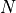matrices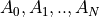, matrix_dot will generate the matrix product between all in the given order, namely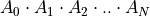.

aesara.tensor.nlinalg.matrix_power(M, n)[source]

Raise a square matrix, M, to the (integer) power n.

This implementation uses exponentiation by squaring which is significantly faster than the naive implementation. The time complexity for exponentiation by squaring is :math: mathcal{O}((n log M)^k)

Parameters: M (TensorVariable) – n (int) –
aesara.tensor.nlinalg.pinv(x, hermitian=False)[source]

Computes the pseudo-inverse of a matrix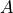.

The pseudo-inverse of a matrix, denoted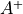, is defined as: “the matrix that ‘solves’ [the least-squares problem]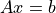,” i.e., if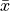is said solution, thenis that matrix such that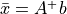.

Note that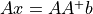, so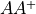is close to the identity matrix. This method is not faster than matrix_inverse. Its strength comes from that it works for non-square matrices. If you have a square matrix though, matrix_inverse can be both more exact and faster to compute. Also this op does not get optimized into a solve op.

aesara.tensor.nlinalg.qr(a, mode='reduced')[source]

Computes the QR decomposition of a matrix. Factor the matrix a as qr, where q is orthonormal and r is upper-triangular.

Parameters: a (array_like, shape (M, N)) – Matrix to be factored. mode ({'reduced', 'complete', 'r', 'raw'}, optional) – If K = min(M, N), then ’reduced’returns q, r with dimensions (M, K), (K, N) ’complete’returns q, r with dimensions (M, M), (M, N) ’r’returns r only with dimensions (K, N) ’raw’returns h, tau with dimensions (N, M), (K,) Note that array h returned in ‘raw’ mode is transposed for calling Fortran. Default mode is ‘reduced’ q (matrix of float or complex, optional) – A matrix with orthonormal columns. When mode = ‘complete’ the result is an orthogonal/unitary matrix depending on whether or not a is real/complex. The determinant may be either +/- 1 in that case. r (matrix of float or complex, optional) – The upper-triangular matrix.
aesara.tensor.nlinalg.svd(a, full_matrices=1, compute_uv=1)[source]

This function performs the SVD on CPU.

Parameters: full_matrices (bool, optional) – If True (default), u and v have the shapes (M, M) and (N, N), respectively. Otherwise, the shapes are (M, K) and (K, N), respectively, where K = min(M, N). compute_uv (bool, optional) – Whether or not to compute u and v in addition to s. True by default. U, V, D matrices
aesara.tensor.nlinalg.tensorinv(a, ind=2)[source]

Aesara utilization of numpy.linalg.tensorinv;

Compute the ‘inverse’ of an N-dimensional array. The result is an inverse for a relative to the tensordot operation tensordot(a, b, ind), i. e., up to floating-point accuracy, tensordot(tensorinv(a), a, ind) is the “identity” tensor for the tensordot operation.

Parameters: a (array_like) – Tensor to ‘invert’. Its shape must be ‘square’, i. e., prod(a.shape[:ind]) == prod(a.shape[ind:]). ind (int, optional) – Number of first indices that are involved in the inverse sum. Must be a positive integer, default is 2. b – a’s tensordot inverse, shape a.shape[ind:] + a.shape[:ind]. ndarray LinAlgError – If a is singular or not ‘square’ (in the above sense).
aesara.tensor.nlinalg.tensorsolve(a, b, axes=None)[source]

Aesara utilization of numpy.linalg.tensorsolve.

Solve the tensor equation a x = b for x. It is assumed that all indices of x are summed over in the product, together with the rightmost indices of a, as is done in, for example, tensordot(a, x, axes=len(b.shape)).

Parameters: a (array_like) – Coefficient tensor, of shape b.shape + Q. Q, a tuple, equals the shape of that sub-tensor of a consisting of the appropriate number of its rightmost indices, and must be such that prod(Q) == prod(b.shape) (in which sense a is said to be ‘square’). b (array_like) – Right-hand tensor, which can be of any shape. axes (tuple of ints, optional) – Axes in a to reorder to the right, before inversion. If None (default), no reordering is done. x ndarray, shape Q LinAlgError – If a is singular or not ‘square’ (in the above sense).
aesara.tensor.nlinalg.trace(X)[source]

Returns the sum of diagonal elements of matrix X.